# Units - math word problems

1. Bar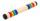Half of the bar is green, the third is yellow and the remaining 40cm is red. How long is a bar?
2. Tour35 people went on a tour and paid 8530, -. Employees pay 165, and family members 310. How many employees and how many family members went to the tour?
3. Good swimmerGood swimmer swims 23 m distance with ten shots. With how many shots he swim to an island located 81 m if still swims at the same speed?
4. Collecting day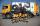At collecting day at school pupils of three classes took total of 1063 kg of paper. Class B collected half more than A Class and class C 55 kg more than B. How many kg of paper collected each class?
5. Cords joining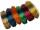Mum needs 6 cords long 360cm long. How many does she need when they are sold at 9 meters and does not want to join it up?
6. Dividing moneyImrich, Daniel and Dezider shared an unknown amount in the ratio 1:2:4, where Dezider received 750 euros more than Imrich and Daniel got half as much as Dezider. Determine an unknown amount of money and determine the amounth that got Imrich, Daniel and De
7. One deciWine 0,375 l costs 2040 CZK. How much does 1 dl cost?
8. Raw meatIn the school cafeteria for one lunch, they prepare 490 servings of 50 g of boiled meat. Meat loses 30% of its weight by cooking. How many kilograms of raw meat must the school canteen prepare for this lunch to cook?
9. Scalene triangleSolve the triangle: A = 50°, b = 13, c = 6
10. Family party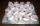Anna has 5 €, Anezka has 4,60 € and for all the money they want to buy desserts for a family party. They decide between cakes and pinwheel: The pinwheel is € 0.40 more expensive than the cake, and the cakes can be bought for all the money by a third more t
11. Find the areaFind the area of the triangle with the given measurements. Round the solution to the nearest hundredth if necessary. A = 50°, b = 30 ft, c = 14 ft
12. GardenThe garden has the shape of a rectangle measuring 19m20cm and 21m60cm. Mr. Novák will fence it. It wants the distance between adjacent pillars to be at least two meters and a maximum of three meters. He would also like the distances between the adjacent pi
13. Three friendsDanica, Lenka and Dalibor have altogether 96 kg. Lenka weighs 75% more than Dalibor and Danica weighs 6 kg more than Dalibor. Determine the weight of Danice, Lenka and Dalibor.
14. Conical areaA right angled triangle has sides a=12 and b=19 in right angle. The hypotenuse is c. If the triangle rotates on the c side as axis, find the volume and surface area of conical area created by this rotation.
15. Two boats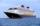The sightseeing boat sail at 25 km / h. The cruise take 6 hours. How long the another boat sails at 60 km/h?
16. Shoes 4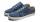Belinda's shoe is 12.45 centimeters long. Felix's shoe is 2.8 centimeters longer than Belinda's shoe. In centimeters, what is the combined length of their two shoes?
17. Drying apples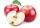By drying, apples lose 85% of their weight. How many kgs of dried apples do we get from 820 kilograms of fresh?
18. PumpingThe tank is filled with a larger pump in 12 hours and with scond pump in 15 hours. For how long does the tank fill when both pumps are switched on at the same time?
19. Resultant forceCalculate mathematically and graphically the resultant of a three forces with a common centre if: F1 = 50 kN α1 = 30° F2 = 40 kN α2 = 45° F3 = 40 kN α3 = 25°
20. Two lands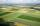The common area of the two neighboring lands is 964 m2. The second land is 77 m2 smaller than twice the size of the first land. Find the areas of each land.

Do you have an interesting mathematical word problem that you can't solve it? Enter it, and we can try to solve it.

To this e-mail address, we will reply solution; solved examples are also published here. Please enter the e-mail correctly and check whether you don't have a full mailbox.

Please do not submit problems from current active competitions such as Mathematical Olympiad, correspondence seminars etc...